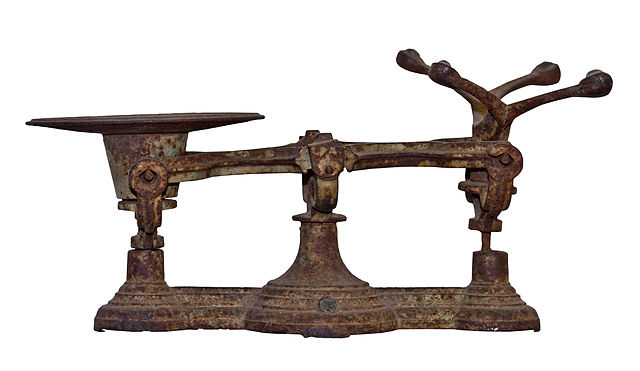Weighing TroubleYou have a weighing balance, which you can place weights on both sides of. You need to measure all weights between 1 and 1000.

For example if you have weights 1 and 3, you can measure test objects of weights 1, 3 or 4. You can also measure objects of weight 2, by placing 3 on one side and 1 on the side which contain the object to be weighed.

What is the minimum number of weights that you would need to be able to measure all (integral) weights from 1 kg to 1000 kg?

×